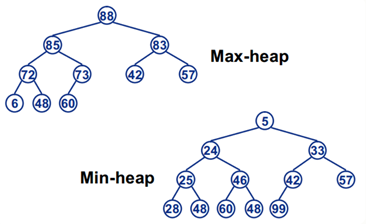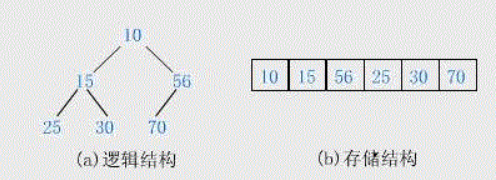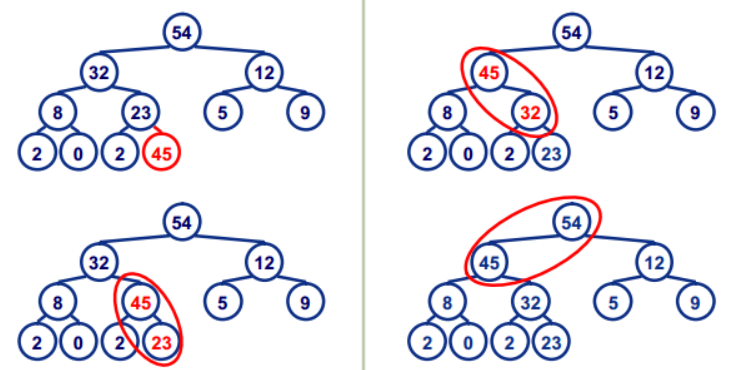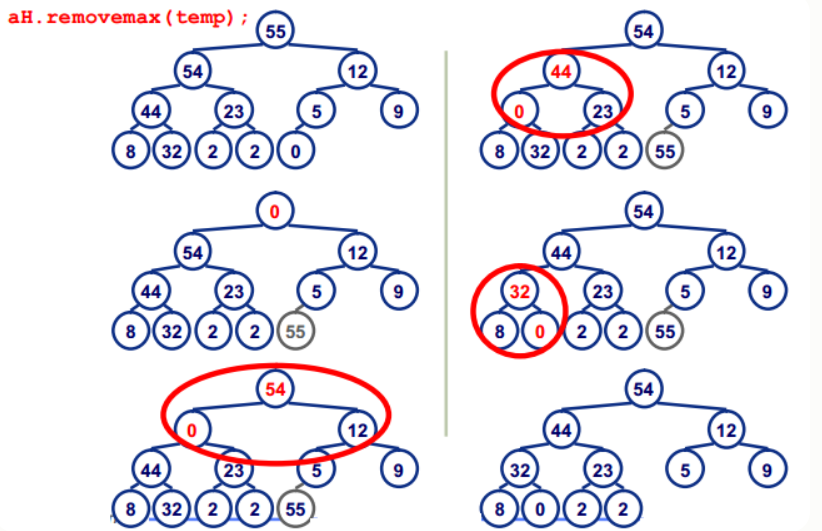# 定義

Heap是一種資料結構具有以下的特點：
1）完全二叉樹；
2）heap中儲存的值是偏序；

Min-heap: 父節點的值小於或等於子節點的值；
Max-heap: 父節點的值大於或等於子節點的值；## 堆的儲存## 堆的核心操作（手寫與系統均有）

heapinsert與heapify
1.heapinsert:加入一個節點，通過上升的方式，依次進行比較，（上升操作），時間複雜度為o（logN）因為樹的深度為logNswap為交換操作

``````private void heapInsert(int[] arr, int index) {

while (arr[index] > arr[(index - 1) / 2]) {

swap(arr, index, (index - 1) / 2);
index = (index - 1) / 2;
}
}
``````

2.heapify：彈出根結點之後的，將剩餘變成堆的操作（下沉操作），因為彈出根結點後，會將陣列最後面的數字頂替為根結點，所以只需將該結點進行下沉操作即可，時間複雜度為o（logN）樹的深度為logNswap為交換操作

``````/**
*
* @param arr 陣列
* @param index 索引
* @param heapSize 堆大小
*/
private void heapify(int[] arr, int index, int heapSize) {

int left = index * 2 + 1;
while (left < heapSize) {

int largest = left + 1 < heapSize && arr[left + 1] > arr[left] ? left + 1 : left;
largest = arr[largest] > arr[index] ? largest : index;
if (largest == index) {

break;
}
swap(arr, largest, index);
index = largest;
left = index * 2 + 1;
}
}
``````

## 堆排序

1.將一個無序陣列變成堆
2.將堆進行排序核心操作（heapfiy，因為如果是大根堆的話，最大值在上方一直，heapfiy即可＋swap（交換）即可）

1.最常用的一種是將陣列依次遍歷，最後一次加入堆中，便可將陣列變成堆，但是此種方法的因為將陣列中所以數進行遍歷 （N），將每個數進行heapinsert（logN），所以時間複雜度為O（N*logN）

``````// O(N*logN)
for (int i = 0; i < arr.length; i++) {

// O(N)
heapInsert(arr, i); // O(logN)
}
``````

2.對此有一種優化方法，從陣列尾部開始遍歷，然後進行下沉，由於是從尾部，那邊可以得到下沉數字一定為有限常數

``````for (int i = arr.length - 1; i >= 0; i--) {

heapify(arr, i, arr.length);
}
``````

``````// 堆排序額外空間複雜度O(1)
public static void heapSort(int[] arr) {

if (arr == null || arr.length < 2) {

return;
}
// O(N*logN)
//		for (int i = 0; i < arr.length; i++) { // O(N)
//			heapInsert(arr, i); // O(logN)
//		}
//O(N)
for (int i = arr.length - 1; i >= 0; i--) {

heapify(arr, i, arr.length);
}
int heapSize = arr.length;
swap(arr, 0, --heapSize);
// O(N*logN)
while (heapSize > 0) {

// O(N)
heapify(arr, 0, heapSize); // O(logN)
swap(arr, 0, --heapSize); // O(1)
}
}
``````

## 手寫解決上述問題優化的堆

``````public static class MyHeap<T> {

private ArrayList<T> heap;
private HashMap<T, Integer> indexMap;
private int heapSize;
private Comparator<? super T> comparator;

public MyHeap(Comparator<? super T> com) {

heap = new ArrayList<>();
indexMap = new HashMap<>();
heapSize = 0;
comparator = com;
}
public boolean isEmpty() {

return heapSize == 0;
}
public int size() {

return heapSize;
}
public boolean contains(T key) {

return indexMap.containsKey(key);
}

public void push(T value) {

indexMap.put(value, heapSize);
heapInsert(heapSize++);
}

public T pop() {

T ans = heap.get(0);
int end = heapSize - 1;
swap(0, end);
heap.remove(end);
indexMap.remove(ans);
heapify(0, --heapSize);
return ans;
}
public void resign(T value) {

int valueIndex = indexMap.get(value);
heapInsert(valueIndex);
heapify(valueIndex, heapSize);
}
private void heapInsert(int index) {

while (comparator.compare(heap.get(index), heap.get((index - 1) / 2)) < 0) {

swap(index, (index - 1) / 2);
index = (index - 1) / 2;
}
}
private void heapify(int index, int heapSize) {

int left = index * 2 + 1;
while (left < heapSize) {

int largest = left + 1 < heapSize && (comparator.compare(heap.get(left + 1), heap.get(left)) < 0)
? left + 1
: left;
largest = comparator.compare(heap.get(largest), heap.get(index)) < 0 ? largest : index;
if (largest == index) {

break;
}
swap(largest, index);
index = largest;
left = index * 2 + 1;
}
}
private void swap(int i, int j) {

T o1 = heap.get(i);
T o2 = heap.get(j);
heap.set(i, o2);
heap.set(j, o1);
indexMap.put(o1, j);
indexMap.put(o2, i);
}
}
``````